# pitch

Estimate fundamental frequency of audio signal

## Syntax

``f0 = pitch(audioIn,fs)``
``f0 = pitch(audioIn,fs,Name,Value)``
``[f0,loc] = pitch(___)``

## Description

example

````f0 = pitch(audioIn,fs)` returns estimates of the fundamental frequency over time for the audio input, `audioIn`, with sample rate `fs`. Columns of the input are treated as individual channels.```

example

````f0 = pitch(audioIn,fs,Name,Value)` specifies options using one or more `Name,Value` pair arguments.```

example

````[f0,loc] = pitch(___)` returns the locations, `loc`, associated with fundamental frequency estimates.```

## Examples

collapse all

Read in an audio signal. Call `pitch` to estimate the fundamental frequency over time.

```[audioIn,fs] = audioread('Hey.ogg'); f0 = pitch(audioIn,fs);```

Listen to the audio signal and plot the signal and pitch. The `pitch` function returns an estimate of the fundamental frequency over time, but the estimate is only valid for regions that are harmonic.

```sound(audioIn,fs) tiledlayout(2,1) nexttile plot(audioIn) xlabel('Sample Number') ylabel('Amplitude') nexttile plot(f0) xlabel('Frame Number') ylabel('Pitch (Hz)')```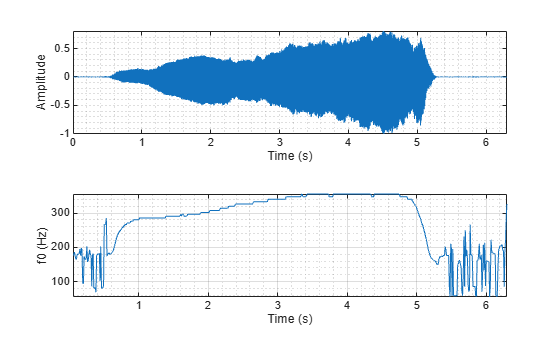Read in an audio signal and extract the pitch.

```[x,fs] = audioread('singing-a-major.ogg'); t = (0:size(x,1)-1)/fs; winLength = round(0.05*fs); overlapLength = round(0.045*fs); [f0,idx] = pitch(x,fs,'Method','SRH','WindowLength',winLength,'OverlapLength',overlapLength); tf0 = idx/fs;```

Listen to the audio and plot the audio and pitch estimations.

```sound(x,fs) figure tiledlayout(2,1) nexttile plot(t,x) ylabel('Amplitude') title('Audio Signal') axis tight nexttile plot(tf0,f0) xlabel('Time (s)') ylabel('Pitch (Hz)') title('Pitch Estimations') axis tight```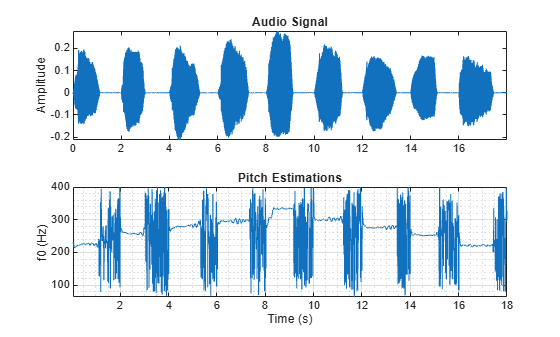The `pitch` function estimates the pitch for overlapped analysis windows. The pitch estimates are only valid if the analysis window has a harmonic component. Call the `harmonicRatio` function using the same window and overlap length used for pitch detection. Plot the audio, pitch, and harmonic ratio.

```hr = harmonicRatio(x,fs,"Window",hamming(winLength,'periodic'),"OverlapLength",overlapLength); figure tiledlayout(3,1) nexttile plot(t,x) ylabel('Amplitude') title('Audio Signal') axis tight nexttile plot(tf0,f0) ylabel('Pitch (Hz)') title('Pitch Estimations') axis tight nexttile plot(tf0,hr) xlabel('Time (s)') ylabel('Ratio') title('Harmonic Ratio') axis tight```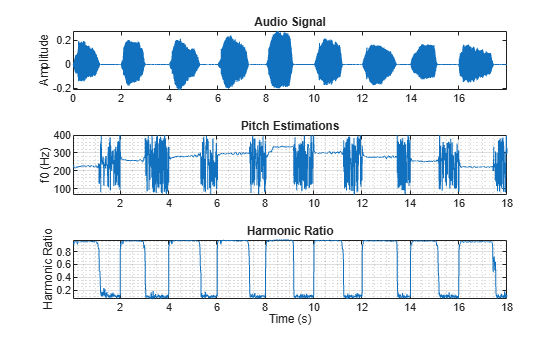Use the harmonic ratio as the threshold for valid pitch decisions. If the harmonic ratio is less than the threshold, set the pitch decision to `NaN`. Plot the results.

```threshold = 0.9; f0(hr < threshold) = nan; figure plot(tf0,f0) xlabel('Time (s)') ylabel('Pitch (Hz)') title('Pitch Estimations') grid on```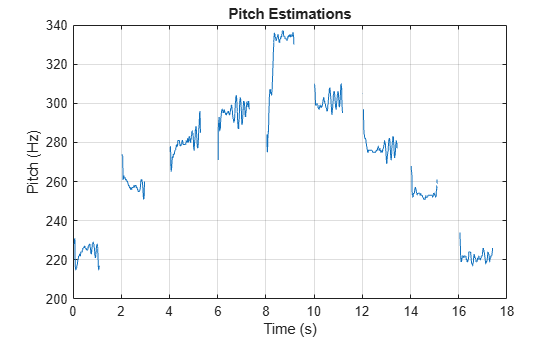Read in an audio signal of a female voice saying "volume up" five times. Listen to the audio.

```[femaleVoice,fs] = audioread('female-volume-up.ogg'); sound(femaleVoice,fs)```

Read in an audio signal of a male voice saying "volume up" five times. Listen to the audio.

```maleVoice = audioread('male-volume-up.ogg'); sound(maleVoice,fs)```

Extract the pitch from both the female and male recordings. Plot histograms of the pitch estimations for the male and female audio recordings. The histograms have a similar shape. This is because the pitch decisions contain results for unvoiced speech and regions of silence.

```f0Female = pitch(femaleVoice,fs); f0Male = pitch(maleVoice,fs); figure numBins = 20; histogram(f0Female,numBins,"Normalization","probability"); hold on histogram(f0Male,numBins,"Normalization","probability"); legend('Female Voice','Male Voice') xlabel('Pitch (Hz)') ylabel('Probability') hold off```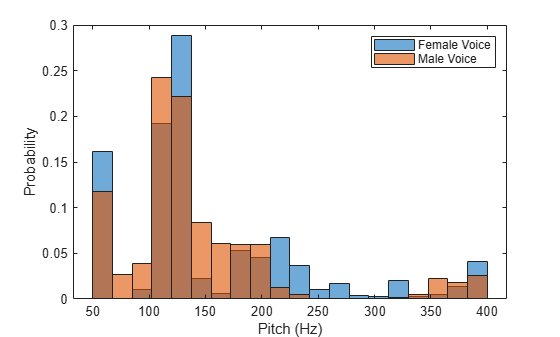Use the `detectSpeech` function to isolate regions of speech in the audio signal and then extract pitch from only those speech regions.

```speechIndices = detectSpeech(femaleVoice,fs); f0Female = []; for ii = 1:size(speechIndices,1) speechSegment = femaleVoice(speechIndices(ii,1):speechIndices(ii,2)); f0Female = [f0Female;pitch(speechSegment,fs)]; end speechIndices = detectSpeech(maleVoice,fs); f0Male = []; for ii = 1:size(speechIndices,1) speechSegment = maleVoice(speechIndices(ii,1):speechIndices(ii,2)); f0Male = [f0Male;pitch(speechSegment,fs)]; end```

Plot histograms of the pitch estimations for the male and female audio recordings. The pitch distributions now appear as expected.

```figure histogram(f0Female,numBins,"Normalization","probability"); hold on histogram(f0Male,numBins,"Normalization","probability"); legend('Female Voice','Male Voice') xlabel('Pitch (Hz)') ylabel('Probability')```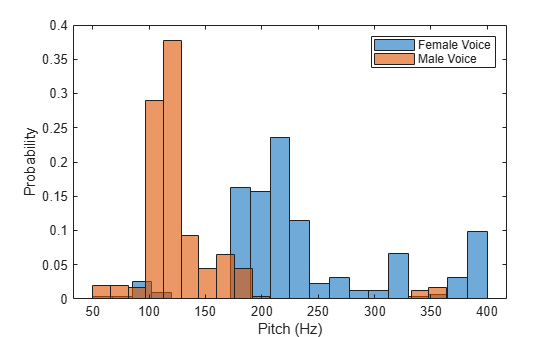Load an audio file of the Für Elise introduction and the sample rate of the audio.

```load FurElise.mat song fs sound(song,fs)```

Call the `pitch` function using the pitch estimate filter (`PEF`), a search range of 50 to 800 Hz, a window duration of 80 ms, an overlap duration of 70 ms, and a median filter length of 10. Plot the results.

```method =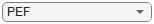"PEF"; range = [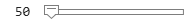50,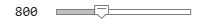800]; % hertz winDur =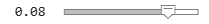0.08; % seconds overlapDur =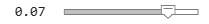0.07; % seconds medFiltLength =10; % frames winLength = round(winDur*fs); overlapLength = round(overlapDur*fs); [f0,loc] = pitch(song,fs, ... 'Method',method, ... 'Range',range, ... 'WindowLength',winLength, ... 'OverlapLength',overlapLength, ... "MedianFilterLength",medFiltLength); t = loc/fs; plot(t,f0) ylabel('Pitch (Hz)') xlabel('Time (s)') grid on```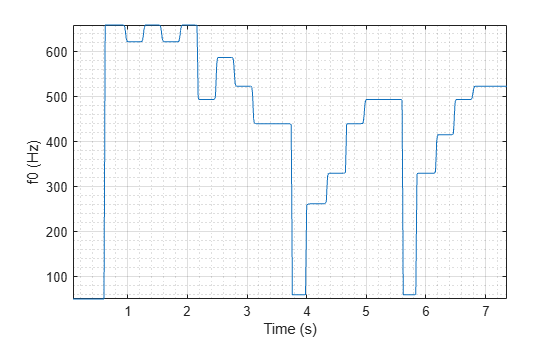Create a `dsp.AudioFileReader` object to read in audio frame-by-frame.

`fileReader = dsp.AudioFileReader('singing-a-major.ogg');`

Create a `voiceActivityDetector` object to detect the presence of voice in streaming audio.

`VAD = voiceActivityDetector;`

While there are unread samples, read from the file and determine the probability that the frame contains voice activity. If the frame contains voice activity, call `pitch` to estimate the fundamental frequency of the audio frame. If the frame does not contain voice activity, declare the fundamental frequency as `NaN`.

```f0 = []; while ~isDone(fileReader) x = fileReader(); if VAD(x) > 0.99 decision = pitch(x,fileReader.SampleRate, ... "WindowLength",size(x,1), ... "OverlapLength",0, ... "Range",[200,340]); else decision = NaN; end f0 = [f0;decision]; end```

Plot the detected pitch contour over time.

```t = linspace(0,(length(f0)*fileReader.SamplesPerFrame)/fileReader.SampleRate,length(f0)); plot(t,f0) ylabel('Fundamental Frequency (Hz)') xlabel('Time (s)') grid on```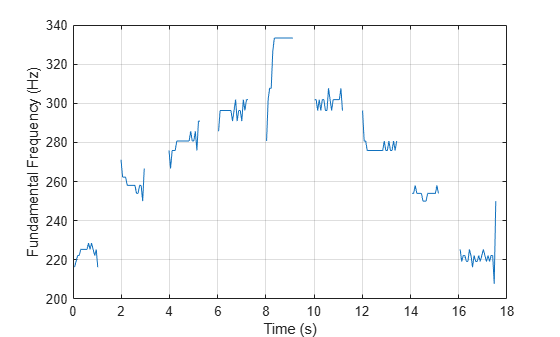The different methods of estimating pitch provide trade-offs in terms of noise robustness, accuracy, optimal lag, and computation expense. In this example, you compare the performance of different pitch detection algorithms in terms of gross pitch error (GPE) and computation time under different noise conditions.

Prepare Test Signals

Load an audio file and determine the number of samples it has. Also load the true pitch corresponding to the audio file. The true pitch was determined as an average of several third-party algorithms on the clean speech file.

```[audioIn,fs] = audioread('Counting-16-44p1-mono-15secs.wav'); numSamples = size(audioIn,1); load TruePitch.mat truePitch```

Create test signals by adding noise to the audio signal at given SNRs. The `mixSNR` function is a convenience function local to this example, which takes a signal, noise, and requested SNR and returns a noisy signal at the request SNR.

```testSignals = zeros(numSamples,4); turbine = audioread('Turbine-16-44p1-mono-22secs.wav'); testSignals(:,1) = mixSNR(audioIn,turbine,20); testSignals(:,2) = mixSNR(audioIn,turbine,0); whiteNoiseMaker = dsp.ColoredNoise('Color','white','SamplesPerFrame',size(audioIn,1)); testSignals(:,3) = mixSNR(audioIn,whiteNoiseMaker(),20); testSignals(:,4) = mixSNR(audioIn,whiteNoiseMaker(),0);```

Save the noise conditions and algorithm names as cell arrays for labeling and indexing.

```noiseConditions = {'Turbine (20 dB)','Turbine (0 dB)','WhiteNoise (20 dB)','WhiteNoise (0 dB)'}; algorithms = {'NCF','PEF','CEP','LHS','SRH'};```

Run Pitch Detection Algorithms

Preallocate arrays to hold pitch decisions for each algorithm and noise condition pair, and the timing information. In a loop, call the `pitch` function on each combination of algorithm and noise condition. Each algorithm has an optimal window length associated with it. In this example, for simplicity, you use the default window length for all algorithms. Use a 3-element median filter to smooth the pitch decisions.

```f0 = zeros(numel(truePitch),numel(algorithms),numel(noiseConditions)); algorithmTimer = zeros(numel(noiseConditions),numel(algorithms)); for k = 1:numel(noiseConditions) x = testSignals(:,k); for i = 1:numel(algorithms) tic f0temp = pitch(x,fs, ... 'Range',[50 300], ... 'Method',algorithms{i}, ... 'MedianFilterLength',3); algorithmTimer(k,i) = toc; f0(1:max(numel(f0temp),numel(truePitch)),i,k) = f0temp; end end```

Compare Gross Pitch Error

Gross pitch error (GPE) is a popular metric when comparing pitch detection algorithms. GPE is defined as the proportion of pitch decisions for which the relative error is higher than a given threshold, traditionally 20% in speech studies. Calculate the GPE and print it to the Command Window.

```idxToCompare = ~isnan(truePitch); truePitch = truePitch(idxToCompare); f0 = f0(idxToCompare,:,:); p = 0.20; GPE = mean( abs(f0(1:numel(truePitch),:,:) - truePitch) > truePitch.*p).*100; for ik = 1:numel(noiseConditions) fprintf('\nGPE (p = %0.2f), Noise = %s.\n',p,noiseConditions{ik}); for i = 1:size(GPE,2) fprintf('- %s : %0.1f %%\n',algorithms{i},GPE(1,i,ik)) end end```
```GPE (p = 0.20), Noise = Turbine (20 dB). ```
```- NCF : 0.9 % - PEF : 0.4 % - CEP : 8.2 % - LHS : 8.2 % - SRH : 6.0 % ```
```GPE (p = 0.20), Noise = Turbine (0 dB). ```
```- NCF : 5.6 % - PEF : 24.5 % - CEP : 11.6 % - LHS : 9.4 % - SRH : 46.8 % ```
```GPE (p = 0.20), Noise = WhiteNoise (20 dB). ```
```- NCF : 0.9 % - PEF : 0.0 % - CEP : 12.9 % - LHS : 6.9 % - SRH : 2.6 % ```
```GPE (p = 0.20), Noise = WhiteNoise (0 dB). ```
```- NCF : 0.4 % - PEF : 0.0 % - CEP : 23.6 % - LHS : 7.3 % - SRH : 1.7 % ```

Calculate the average time it takes to process one second of data for each of the algorithms and print the results.

```aT = sum(algorithmTimer)./((numSamples/fs)*numel(noiseConditions)); for ik = 1:numel(algorithms) fprintf('- %s : %0.3f (s)\n',algorithms{ik},aT(ik)) end```
```- NCF : 0.035 (s) - PEF : 0.112 (s) - CEP : 0.037 (s) - LHS : 0.049 (s) - SRH : 0.095 (s) ```

## Input Arguments

collapse all

Audio input signal, specified as a vector or matrix. The columns of the matrix are treated as individual audio channels.

Data Types: `single` | `double`

Sample rate of the input signal in Hz, specified as a positive scalar.

The sample rate must be greater than or equal to twice the upper bound of the search range. Specify the search range using the `Range` name-value pair.

Data Types: `single` | `double`

### Name-Value Arguments

Specify optional comma-separated pairs of `Name,Value` arguments. `Name` is the argument name and `Value` is the corresponding value. `Name` must appear inside quotes. You can specify several name and value pair arguments in any order as `Name1,Value1,...,NameN,ValueN`.

Example: `pitch(audioIn,fs,'Range',[50,150],'Method','PEF')`

Search range for pitch estimates, specified as the comma-separated pair consisting of 'Range' and a two-element row vector with increasing positive integer values. The function searches for a best estimate of the fundamental frequency within the upper and lower band edges specified by the vector, according to the algorithm specified by `Method`. The range is inclusive and units are in Hz.

Valid values for the search range depend on the sample rate, `fs`, and on the values of `WindowLength` and `Method`:

MethodMinimum RangeMaximum Range
'`NCF`'```fs/WindowLength < Range(1)``````Range(2) < fs/2```
'`PEF`'`10 < Range(1)````Range(2) < min(4000,fs/2)```
'`CEP`'```fs/(2^nextpow2(2*WindowLength-1)) < Range(1)``````Range(2) < fs/2```
'`LHS`'`1 < Range(1)````Range(2) < fs/5 - 1```
'`SRH`'`1 < Range(1)````Range(2) < fs/5 - 1```

Data Types: `single` | `double`

Number of samples in the analysis window, specified as the comma-separated pair consisting of '`WindowLength`' and an integer in the range [1, min(size(`audioIn`,1), 192000)]. Typical analysis windows are in the range 20–100 ms. The default window length is 52 ms.

Data Types: `single` | `double`

Number of samples of overlap between adjacent analysis windows, specified as the comma-separated pair consisting of '`OverlapLength`' and an integer in the range (`-inf`,`WindowLength`). A negative overlap length indicates non-overlapping analysis windows.

Data Types: `single` | `double`

Method used to estimate pitch, specified as the comma-separated pair consisting of '`Method`' and `'NCF'`, `'PEF'`,`'CEP'`, `'LHS'`, or `'SRH'`. The different methods of calculating pitch provide trade-offs in terms of noise robustness, accuracy, and computation expense. The algorithms used to calculate pitch are based on the following papers:

• `'NCF'` –– Normalized Correlation Function 

• `'PEF'` –– Pitch Estimation Filter . The function does not use the amplitude compression described by the paper.

• `'CEP'` –– Cepstrum Pitch Determination 

• `'LHS'` –– Log-Harmonic Summation 

• `'SRH'` –– Summation of Residual Harmonics 

Data Types: `char` | `string`

Median filter length used to smooth pitch estimates over time, specified as the comma-separated pair consisting of '`MedianFilterLength`' and a positive integer. The default, `1`, corresponds to no median filtering. Median filtering is a postprocessing technique used to remove outliers while estimating pitch. The function uses `movmedian` after estimating the pitch using the specified `Method`.

Data Types: `single` | `double`

## Output Arguments

collapse all

Estimated fundamental frequency, in Hz, returned as a scalar, vector, or matrix. The number of rows returned depends on the values of the `WindowLength` and `OverlapLength` name-value pairs, and on the input signal size. The number of columns (channels) returned depends on the number of columns of the input signal size.

Data Types: `single` | `double`

Locations associated with fundamental frequency estimations, returned as a scalar, vector, or matrix the same size as `f0`.

Fundamental frequency is estimated locally over a region of `WindowLength` samples. The values of `loc` correspond to the most recent sample (largest sample number) used to estimate fundamental frequency.

Data Types: `single` | `double`

## Algorithms

The `pitch` function segments the audio input according to the `WindowLength` and `OverlapLength` arguments. The fundamental frequency is estimated for each frame. The locations output, `loc` contains the most recent samples (largest sample numbers) of the corresponding frame.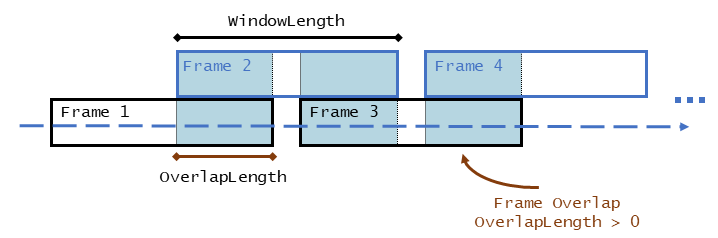For a description of the algorithms used to estimate the fundamental frequency, consult the corresponding references:

• `'NCF'` –– Normalized Correlation Function 

• `'PEF'` –– Pitch Estimation Filter . The function does not use the amplitude compression described by the paper.

• `'CEP'` –– Cepstrum Pitch Determination 

• `'LHS'` –– Log-Harmonic Summation 

• `'SRH'` –– Summation of Residual Harmonics 

 Atal, B.S. "Automatic Speaker Recognition Based on Pitch Contours." The Journal of the Acoustical Society of America. Vol. 52, No. 6B, 1972, pp. 1687–1697.

 Gonzalez, Sira, and Mike Brookes. "A Pitch Estimation Filter robust to high levels of noise (PEFAC)." 19th European Signal Processing Conference. Barcelona, 2011, pp. 451–455.

 Noll, Michael A. "Cepstrum Pitch Determination." The Journal of the Acoustical Society of America. Vol. 31, No. 2, 1967, pp. 293–309.

 Hermes, Dik J. "Measurement of Pitch by Subharmonic Summation." The Journal of the Acoustical Society of America. Vol. 83, No. 1, 1988, pp. 257–264.

 Drugman, Thomas, and Abeer Alwan. "Joint Robust Voicing Detection and Pitch Estimation Based on Residual Harmonics." Proceedings of the Annual Conference of the International Speech Communication Association, INTERSPEECH. 2011, pp. 1973–1976.

## SupportGet trial now